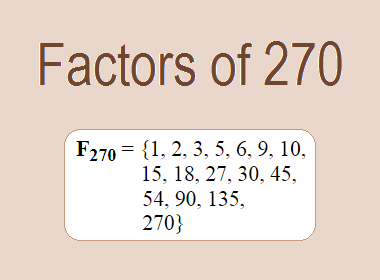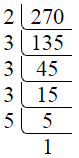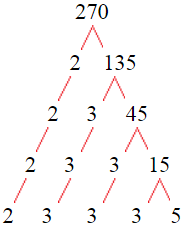# Factors of 270The factors of 270 are 1, 2, 3, 5, 6, 9, 10, 15, 18, 27, 30, 45, 54, 90, 135, and 270 i.e. F270 = {1, 2, 3, 5, 6, 9, 10, 15, 18, 27, 30, 45, 54, 90, 135, 270}. The factors of 270 are all the numbers that can divide 270 without leaving a remainder.

We can check if these numbers are factors of 270 by dividing 270 by each of them. If the result is a whole number, then the number is a factor of 270. Let's do this for each of the numbers listed above:

·        1 is a factor of 270 because 270 divided by 1 is 270.

·        2 is a factor of 270 because 270 divided by 2 is 135.

·        3 is a factor of 270 because 270 divided by 3 is 90.

·        5 is a factor of 270 because 270 divided by 5 is 54.

·        6 is a factor of 270 because 270 divided by 6 is 45.

·        9 is a factor of 270 because 270 divided by 9 is 30.

·        10 is a factor of 270 because 270 divided by 10 is 27.

·        15 is a factor of 270 because 270 divided by 15 is 18.

·        18 is a factor of 270 because 270 divided by 18 is 15.

·        27 is a factor of 270 because 270 divided by 27 is 10.

·        30 is a factor of 270 because 270 divided by 30 is 9.

·        45 is a factor of 270 because 270 divided by 45 is 6.

·        54 is a factor of 270 because 270 divided by 54 is 5.

·        90 is a factor of 270 because 270 divided by 90 is 3.

·        135 is a factor of 270 because 270 divided by 135 is 2.

·        270 is a factor of 270 because 270 divided by 270 is 1.

## How to Find Factors of 270?

1 and the number itself are the factors of every number. So, 1 and 270 are two factors of 270. To find the other factors of 270, we can start by dividing 270 by the numbers between 1 and 270. If we divide 270 by 2, we get a remainder of 0. Therefore, 2 is a factor of 270. If we divide 270 by 3, we get a remainder of 0. Therefore, 3 is also a factor of 270.

Next, we can check if 4 is a factor of 270. If we divide 270 by 4, we get a remainder of 2. Therefore, 4 is not a factor of 270. We can continue this process for all the possible factors of 270.

Through this process, we can find that the factors of 270 are 1, 2, 3, 5, 6, 9, 10, 15, 18, 27, 30, 45, 54, 90, 135, and 270. These are the only numbers that can divide 270 without leaving a remainder.

********************

********************

## Properties of the Factors of 270

The factors of 270 have some interesting properties. One of the properties is that the sum of the factors of 270 is equal to 720. We can see this by adding all the factors of 270 together:

1 + 2 + 3 + 5 + 6 + 9 + 10 + 15 + 18 + 27 + 30 + 45 + 54 + 90 + 135 + 270 = 720

Another property of the factors of 270 is that the prime factors of 270 are 2, 3, and 5 only.

## Applications of the Factors of 270

The factors of 270 have several applications in mathematics. One of the applications is in finding the highest common factor (HCF) of two or more numbers. The HCF is the largest factor that two or more numbers have in common. For example, to find the HCF of 270 and 120, we need to find the factors of both numbers and identify the largest factor they have in common. The factors of 270 are 1, 2, 3, 5, 6, 9, 10, 15, 18, 27, 30, 45, 54, 90, 135, and 270. The factors of 120 are 1, 2, 3, 5, 6, 8, 10, 12, 15, 20, 24, 30, 40, 60, and 120. The largest factor that they have in common is 30. Therefore, the HCF of 270 and 120 is 30.

Another application of the factors of 270 is in prime factorization. Prime factorization is the process of expressing a number as the product of its prime factors. The prime factors of 270 are 2, 3, and 5, since these are the only prime numbers that can divide 270 without leaving a remainder. Therefore, we can express 270 as:

270 = 2 × 3 × 3 × 3 × 5

We can do prime factorization by division and factor tree method also. Here is the prime factorization of 270 by division method,270 = 2 × 3 × 3 × 3 × 5

Here is the prime factorization of 270 by the factor tree method,270 = 2 × 3 × 3 × 3 × 5

## Conclusion

The factors of 270 are the numbers that can divide 270 without leaving a remainder. The factors of 270 are 1, 2, 3, 5, 6, 9, 10, 15, 18, 27, 30, 45, 54, 90, 135, and 270. The factors of 270 have some interesting properties, such as having a sum of 720. The factors of 270 have several applications in mathematics, such as finding the highest common factor and prime factorization.